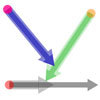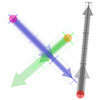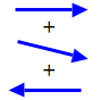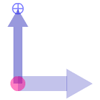Search by Topic

Resources tagged with Complex analysis similar to Impedance Can Be Complex!:

Filter by: Content type:
Age range:
Challenge level:

There are 5 results

Broad Topics > Calculus > Complex analysisImpedance Can Be Complex!

Age 16 to 18 Challenge Level:

Put your complex numbers and calculus to the test with this impedance calculation.Arrow Arithmetic 2

Age 14 to 16 Challenge Level:

Introduces the idea of a twizzle to represent number and asks how one can use this representation to add and subtract geometrically.Arrow Arithmetic 3

Age 14 to 16 Challenge Level:

How can you use twizzles to multiply and divide?Arrow Arithmetic 1

Age 14 to 16 Challenge Level:

The first part of an investigation into how to represent numbers using geometric transformations that ultimately leads us to discover numbers not on the number line.Twizzle Arithmetic

Age 14 to 16 Challenge Level:

Arrow arithmetic, but with a twist.